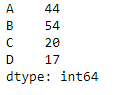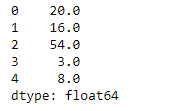# Python | Pandas dataframe.max()

• Last Updated : 19 Nov, 2018

Python is a great language for doing data analysis, primarily because of the fantastic ecosystem of data-centric python packages. Pandas is one of those packages and makes importing and analyzing data much easier.

Pandas` dataframe.max()` function returns the maximum of the values in the given object. If the input is a series, the method will return a scalar which will be the maximum of the values in the series. If the input is a dataframe, then the method will return a series with maximum of values over the specified axis in the dataframe. By default the axis is the index axis.

Syntax: DataFrame.max(axis=None, skipna=None, level=None, numeric_only=None, **kwargs)

Parameters :
axis : {index (0), columns (1)}
skipna : Exclude NA/null values when computing the result
level : If the axis is a MultiIndex (hierarchical), count along a particular level, collapsing into a Series
numeric_only : Include only float, int, boolean columns. If None, will attempt to use everything, then use only numeric data. Not implemented for Series.

Returns : max : Series or DataFrame (if level specified)

Example #1: Use `max()` function to find the maximum value over the index axis.

 `# importing pandas as pd ` `import` `pandas as pd ` ` `  `# Creating the dataframe  ` `df ``=` `pd.DataFrame({``"A"``:[``12``, ``4``, ``5``, ``44``, ``1``], ` `                   ``"B"``:[``5``, ``2``, ``54``, ``3``, ``2``], ` `                   ``"C"``:[``20``, ``16``, ``7``, ``3``, ``8``],  ` `                   ``"D"``:[``14``, ``3``, ``17``, ``2``, ``6``]}) ` ` `  `# Print the dataframe ` `df `Let’s use the `dataframe.max()` function to find the maximum value over the index axis

 `# Even if we do not specify axis = 0,  ` `# the method will return the max over ` `# the index axis by default ` `df.``max``(axis ``=` `0``) `

Output :Example #2: Use `max()` function on a dataframe which has `Na` values. Also find the maximum over the column axis.

 `# importing pandas as pd ` `import` `pandas as pd ` ` `  `# Creating the dataframe  ` `df ``=` `pd.DataFrame({``"A"``:[``12``, ``4``, ``5``, ``None``, ``1``],  ` `                   ``"B"``:[``7``, ``2``, ``54``, ``3``, ``None``], ` `                   ``"C"``:[``20``, ``16``, ``11``, ``3``, ``8``], ` `                   ``"D"``:[``14``, ``3``, ``None``, ``2``, ``6``]}) ` ` `  `# skip the Na values while finding the maximum ` `df.``max``(axis ``=` `1``, skipna ``=` `True``) `

Output :My Personal Notes arrow_drop_up
Recommended Articles
Page :